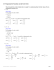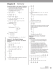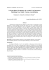# Test 2 Review 1

## Transcription

Test 2 Review 1
```UNIT 2 REVIEW 1
1
NAME: ________________________________
1. Which of the following is a dilation?
A) T ( x, y) − − − − > ( x − 4, y + 3)
B) T ( x, y ) − − − − > ( y, x)
C) T ( x, y) − − − − > (2 x, 2 y)
D) T ( x, y) − − − − > (5x,3 y )
2. Given the original figure, which of the following is a dilation?
A)
Original
B)
C)
D)
3. Which of the following scale factors is an enlargement?
A) 500 : 50
4. If we D
O,−
1
2
Original
B) 0.01 : 0.1
C) 7 : 3.5
D) 0.1 : 0.01
C)
D)
then the correct diagram would be:
A)
B)
m
O
m'
m
O
m
O
m
m
m'
m'
O
O
5. Determine the scale factor of the given dilation from point O?
B
B'
A) 1 : 2
B) 2 : 1
C) 2 : 5
D) 5 : 2
5 cm
2.5 cm
O
C'
C
6. Which of the following is not a similarity transformation?
A) Rotation
B) Reflection
C) Stretch
D) Dilation
7. Which of the following would be the criterion for establishing similarity in the two
triangles?
o
o
•
A) AA
B) SAS
C) SSS
D) Not enough info or not similar
•
UNIT 2 REVIEW 1
8. In the given diagram, which of the following statement is NOT true:
A)
AB AC
AB DE
=
=
B)
DE DF
EF BC
C)
AB DE
=
BC EF
D)
G
GB GE
=
GA GD
D
A
E
B
F
C
9. The geometric mean of 4 and 16 is:
A) 8
B) 10
C) 12
D) cannot be determined
10. The value for x is:
o
x
o
A) 16
B) 16 2
C) 16 3
D) 32
16
11. The value for x is:
x
4
A) 2
B) 2 2
C) 4 3
D) 2 3
60°
12. The ratio of the short leg to the hypotenuse in a 30° right triangle is:
A) 1 : 2
B) 1 :
C) 2 : 1
3
D) 1 :
2
13. If cos Ɵ = sin ß then the two angles must be:
A) supplementary
14. The ratio
A) sin ∠C
B) complementary
C) a linear pair
A
5
represent the which relationship:
13
B) sin ∠B
C) tan ∠C
12 cm
D) cos ∠C
C
5 cm
B
13 cm
UNIT 2 REVIEW 1
15. Tommy has caught his kite at the top of a 16 ft tree. From where
Tommy is standing the elevation to the top of the tree is 29°, what is the
length of string (round to the nearest foot)??
A) 33 ft
B) 29 ft
C) 18 ft
D) 8 ft
16. If in ∆ABC & ∆GHY, ∠C ≅ ∠Y & ∠B ≅ ∠Η then ∆ABC ∼ ∆GHY.
T or F
17. The hypotenuse is always opposite the right angle.
T or F
18. If ∆ABC ∼ ∆DEF ∼ ∆MNP, then ∠B ≅ ∠N
T or F
19. DO ,2 ( AB) = A ' B ' , then AB || A ' B ' .
T or F
20. Given DO ,3 P ( −1, 0) then P '( −3, 3)
T or F
21. sin (x – 5) = cos (4x + 10) when x =17.
T or F
Solve for the unknown.
22. sin (x + 18°) = cos (45°)
23. sin (2x – 15°) = cos (x – 12°)
24. A loading ramp is 21 m long with a height of 7 m. What is the angle
of incline that the ramp forms with the ground? (2 decimal places)
UNIT 2 REVIEW 1
25. The landing pad is 125 ft away from the man. When looking up at the
helicopter that is hovering over the landing pad he sees it at an angle of elevation
of 50°. What is the altitude of the helicopter (round to the nearest foot)?
26. A flagpole is at the top of a building. 250 ft from the base of the building, the
angle of elevation of the top of the pole is 50° and the angle of elevation of the
bottom of the pole is 48°. Determine the length of the flagpole (to the nearest foot).
27. From an apartment window 20 ft above the ground in the shorter
building, the angle of depression of the base of a nearby tower is 12°
and the angle of elevation of the top of the tower is 40°. Find the
height of the nearby building (to the nearest foot).
UNIT 2 REVIEW 1
Find the missing values. (If not a whole number, round to 2 decimal places)
28.
29.
30.
z
x
12
y
x
x
8
8
8
z
8
9
y
y
z
x = ________ y = _________
z = ________
x = ________ y = _________
z = ________
x = ________ y = _________
z = ________
Determine the Geometric Mean of the two given numbers. (Exact Answers Only)
31.
2 and 8
32.
12 and 27
33.
20 and 25
34.
10 and 16
Are the following pairs of triangles similar? If they are, then name their similarity criteria. (SSS, SAS, AA)
35. Yes / No __________
0
4
36. Yes / No __________
5
o
6.25
5
o
37. Yes / No __________
UNIT 2 REVIEW 1
Determine the sequence of similarity transformations that map one figure onto the other thus establishing that
the two figures are similar.
38. Determine two similarity transformations that
would map ∆OBC onto ∆OLK.
39. Determine two similarity transformations that
_________________ followed by _________________
_________________ followed by _________________
A'
Given the dilation centered at O,
A
40. Is this an enlargement or reduction?
How did you determine it?
41. If AB = 3.5 cm and A’B’ = 10.5 cm,
what is the scale factor of the dilation?
O
B
B'
UNIT 2 REVIEW 1
42. Center of dilation is G.
Scale Factor 3
G (0,3)
A (5,9)
Determine the slope of GA from G ( x1 , y1 ) to
A ( x2 , y2 )
m=
y2 − y1
x2 − x1
Determine A’.
Find the values for the missing variables.
43.
44.
15.75
x
3
18
x
o
o
8
x+1
4
x = __________
x = __________
UNIT 2 REVIEW 1
45. When looking at a trigonometry table Sarah notices that the Sine and Cosine ratios are the same value for
the 45° right triangle. Explain why that happened.
Solve for the missing information. (Round all final answers to 2 decimals places)
46.
47.
7 cm
θ°
6 cm
x
10 cm
24°
x ≈ ____________
48.
Ɵ = ____________
49.
8 cm
5 cm
θ°
12 cm
Ɵ = ____________
x
44°
x ≈ ____________
UNIT 2 REVIEW 1
50. Solve – The ratio of two supplementary angles is 3:5. Find the measure of each angle. (2 points)
_______ & _______
51. Prove the following relationships.
a) GIVEN:
PROVE:
QR || ST
RP QR
=
SP ST
STATEMENT
R
Q
P
S
T
REASON
52. Use a compass and a straightedge to construct DO ,−1 ( ∆ADB )
A
O
C
B
UNIT 2 REVIEW 1
UNIT 2 REVIEW 1
1. C
2. B
3. B
4. A
5.B
6.C
7.A
8.B
9.A
10.B
13. B
14.A
15.A
16.T
17.T
18.T
19.T
20. F
21. T
22. x=27
24. sin −1 (7 / 21) = θ
θ =19.47°
26. tan 50° =
x
x = 297.94 ft
250
27. tan12° =
20
x = 94.09 ft
x
11.D
12.A
23. x=39
25. 149 ft
tan 48° =
tan 40° =
y
y = 277.65 ft 297.94 − 277.65 = 20.29 ft = 20 ft
250
y
y = 78.95 ft 78.95 + 20 = 98.95 ft = 99 ft
94.09
28. x = 14.42, y = 5.33, z = 9.61
7.11, z = 12.04
29. x = 11.31, y = 8, z = 11.31
30. x = 10.70, y =
31. 4
34. 4 10
37. Yes SAS
32. 18
33. 10 5
35. Yes SAS
36. No
38. various answers such as DO,2 (∆LKO) followed by R O, -90 (∆L’K’O’)
39. VA
Ry-axis (HTOR) fd by DO,3 (H’T’O’R’)
6
41. 3
42. m= 5 ; A’(15, 21)
40. Enlargement; Image is further away from center
43. X=3
44. X=7
45. creates a right isosceles triangle so the opp and adj sides are equal.
48. 22.62°
49. 11.52 cm 50. 67.5 & 112.5
51.
STATEMENT
QR || ST
REASON
Given
52.
∠Q ≅ ∠T
|| --> Alt. Interior ∠ ≅
∠S ≅ ∠R
|| --> Alt. Interior ∠ ≅
∆QPR ∼ ∆TPS
AA
RP QR
=
SP ST
∆QPR ∼ ∆TPS
(prop. sides of ∼ ∆)
46. 2.67 cm
47. 45.57°
```

### Test 2 Review 2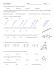### AS Entrance Examination Sample Paper Mathematics Instructions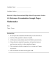### Calculus I Final, Sample### Worksheet - Multi-Step Trigonometry Problem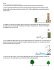### Problem Solving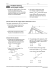### Worksheet: Rotation and Dilation Name 1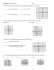### Practice - buskemath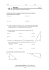### Document 6539622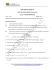### NTSE PHYSICS SAMPLE PAPER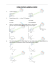### File - HHS Pre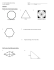### MATH 307: Problem Set #6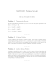### Sample Solutions to Quiz 3 for MATH3270A − y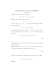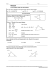### installation of an angle board * * * thermopian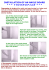### Review Sheet for Second Midterm Mathematics 1300, Calculus 1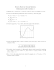### Time : 120 Minutes Max. Marks : 60 GENERAL INSTRUCTIONS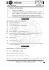### 1.6 Exam advice and sample questions for Chapter 1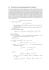### Angles of Elevation and Depression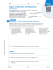### 11.2 Proving Figures are Similar Using Transformations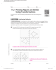### 4-3 Trigonometric Functions on the Unit Circle